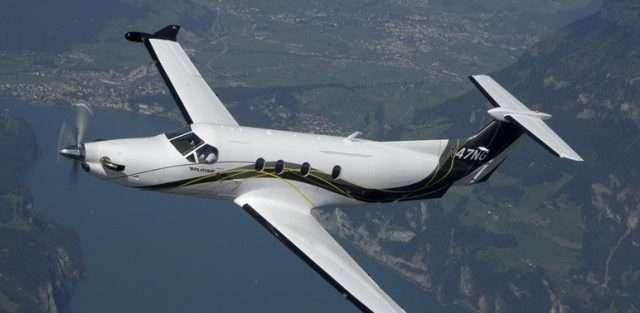# Standard Rate TurnStandard rate turn refers to the act of maneuvering an aircraft at a standard rate of three degrees per second. There are 360 degrees in a circle, so it would take an air charter pilot exactly two minutes to complete a full turn at the standard rate using a constant-bank level turn.

By standardizing the rate of turn, it helps pilots and air traffic controllers know what the other is doing. Standard rate turns are used in:

• Holding patterns
• Tracking
• Approaches
• Departures
• Vectoring

## The Effect of Airspeed on Standard Rate Turns

The airspeed of an air charter affects it ability to complete a standard rate turn. It might seem counterintuitive, but the faster a plane travels, the higher bank angle it requires to complete the turn. By travelling faster, the aircraft is increasing the turn radius, and decreasing the rate of turn.

Of course, increasing the bank angle beyond a certain point isn’t advisable. Therefore, the ICAO typically limits the maximum bank angle of faster aircraft to 25 degrees when they are in a holding pattern.

Alternatively, in cases where aircraft are flying in excess of 250 knots, the air charter pilot can follow a rate half turn of 1.5 degrees per second. Using this method, it would take twice as long, or four minutes, to complete a full turn.

In order to accurately navigate a standard rate turn, aircraft are equipped with either a turn coordinator or a turn and bank indicator (also known as a turn and slip indicator).